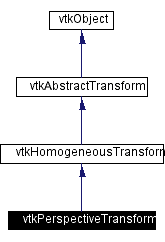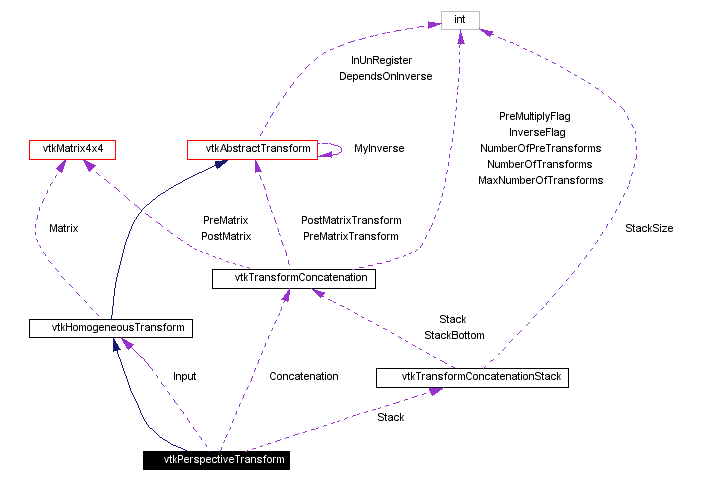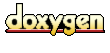Main Page   Class Hierarchy   Alphabetical List   Compound List   File List   Compound Members   File Members   Related Pages

# vtkPerspectiveTransform Class Reference

describes a 4x4 matrix transformation. More...

`#include <vtkPerspectiveTransform.h>`

Inheritance diagram for vtkPerspectiveTransform:[legend]
Collaboration diagram for vtkPerspectiveTransform:[legend]
List of all members.

## Public Methods

virtual const char * GetClassName ()
virtual int IsA (const char *type)
void PrintSelf (ostream &os, vtkIndent indent)
void Identity ()
void Inverse ()
void AdjustViewport (double oldXMin, double oldXMax, double oldYMin, double oldYMax, double newXMin, double newXMax, double newYMin, double newYMax)
void AdjustZBuffer (double oldNearZ, double oldFarZ, double newNearZ, double newFarZ)
void Ortho (double xmin, double xmax, double ymin, double ymax, double znear, double zfar)
void Frustum (double xmin, double xmax, double ymin, double ymax, double znear, double zfar)
void Perspective (double angle, double aspect, double znear, double zfar)
void Shear (double dxdz, double dydz, double zplane)
void Stereo (double angle, double focaldistance)
void SetupCamera (const double position, const double focalpoint, const double viewup)
void Translate (double x, double y, double z)
void Translate (const double x)
void Translate (const float x)
void RotateWXYZ (double angle, double x, double y, double z)
void RotateWXYZ (double angle, const double axis)
void RotateWXYZ (double angle, const float axis)
void RotateX (double angle)
void RotateY (double angle)
void RotateZ (double angle)
void Scale (double x, double y, double z)
void Scale (const double s)
void Scale (const float s)
void SetMatrix (vtkMatrix4x4 *matrix)
void SetMatrix (const double elements)
void Concatenate (vtkMatrix4x4 *matrix)
void Concatenate (const double elements)
void Concatenate (vtkHomogeneousTransform *transform)
void PreMultiply ()
void PostMultiply ()
void Push ()
void Pop ()
void SetInput (vtkHomogeneousTransform *input)
vtkHomogeneousTransformGetInput ()
vtkAbstractTransformMakeTransform ()
int CircuitCheck (vtkAbstractTransform *transform)
unsigned long GetMTime ()

## Static Public Methods

vtkPerspectiveTransform * New ()
int IsTypeOf (const char *type)
vtkPerspectiveTransform * SafeDownCast (vtkObject *o)

## Protected Methods

vtkPerspectiveTransform ()
~vtkPerspectiveTransform ()
vtkPerspectiveTransform (const vtkPerspectiveTransform &t)
void operator= (const vtkPerspectiveTransform &)
void InternalDeepCopy (vtkAbstractTransform *t)
void InternalUpdate ()

## Protected Attributes

vtkHomogeneousTransformInput
vtkTransformConcatenationConcatenation
vtkTransformConcatenationStackStack

## Detailed Description

describes a 4x4 matrix transformation.

Date:
2000/12/10 20:08:14
Revision:
1.21

A vtkPerspectiveTransform can be used to describe the full range of homogeneous transformations. It was designed in particular to describe a camera-view of a scene.

The order in which you set up the display coordinates (via AdjustZBuffer() and AdjustViewport()), the projection (via Perspective(), Frustum(), or Ortho()) and the camera view (via SetupCamera()) are important. If the transform is in PreMultiply mode, which is the default, set the Viewport and ZBuffer first, then the projection, and finally the camera view. Once the view is set up, the Translate and Rotate methods can be used to move the camera around in world coordinates. If the Oblique() or Stereo() methods are used, they should be called just before SetupCamera().

In PostMultiply mode, you must perform all transformations in the opposite order. This is necessary, for example, if you already have a perspective transformation set up but must adjust the viewport. Another example is if you have a view transformation, and wish to perform translations and rotations in the camera's coordinate system rather than in world coordinates.

The SetInput and Concatenate methods can be used to create a transformation pipeline with vtkPerspectiveTransform. See vtkTransform for more information on the transformation pipeline.

vtkGeneralTransform vtkTransform vtkMatrix4x4 vtkCamera
Examples:
vtkPerspectiveTransform (examples)

Definition at line 79 of file vtkPerspectiveTransform.h.

## Constructor & Destructor Documentation

 vtkPerspectiveTransform::vtkPerspectiveTransform ( ) ` [protected]`

 vtkPerspectiveTransform::~vtkPerspectiveTransform ( ) ` [protected]`

 vtkPerspectiveTransform::vtkPerspectiveTransform ( const vtkPerspectiveTransform & t ) ` [protected]`

## Member Function Documentation

 vtkPerspectiveTransform* vtkPerspectiveTransform::New ( ) ` [static]`
 Create an object with Debug turned off, modified time initialized to zero, and reference counting on. Reimplemented from vtkObject.

 virtual const char* vtkPerspectiveTransform::GetClassName ( ) ` [virtual]`
 Return the class name as a string. This method is defined in all subclasses of vtkObject with the vtkTypeMacro found in vtkSetGet.h. Reimplemented from vtkHomogeneousTransform.

 int vtkPerspectiveTransform::IsTypeOf ( const char * type ) ` [static]`
 Return 1 if this class type is the same type of (or a subclass of) the named class. Returns 0 otherwise. This method works in combination with vtkTypeMacro found in vtkSetGet.h. Reimplemented from vtkHomogeneousTransform.

 virtual int vtkPerspectiveTransform::IsA ( const char * type ) ` [virtual]`
 Return 1 if this class is the same type of (or a subclass of) the named class. Returns 0 otherwise. This method works in combination with vtkTypeMacro found in vtkSetGet.h. Reimplemented from vtkHomogeneousTransform.

 vtkPerspectiveTransform* vtkPerspectiveTransform::SafeDownCast ( vtkObject * o ) ` [static]`
 Will cast the supplied object to vtkObject* is this is a safe operation (i.e., a safe downcast); otherwise NULL is returned. This method is defined in all subclasses of vtkObject with the vtkTypeMacro found in vtkSetGet.h. Reimplemented from vtkHomogeneousTransform.

 void vtkPerspectiveTransform::PrintSelf ( ostream & os, vtkIndent indent ) ` [virtual]`
 Methods invoked by print to print information about the object including superclasses. Typically not called by the user (use Print() instead) but used in the hierarchical print process to combine the output of several classes. Reimplemented from vtkHomogeneousTransform.

 void vtkPerspectiveTransform::Identity ( ) ` [inline]`
 Set this transformation to the identity transformation. If the transform has an Input, then the transformation will be reset so that it is the same as the Input. Reimplemented from vtkAbstractTransform. Definition at line 89 of file vtkPerspectiveTransform.h.

 void vtkPerspectiveTransform::Inverse ( ) ` [inline, virtual]`
 Invert the transformation. This will also set a flag so that the transformation will use the inverse of its Input, if an Input has been set. Reimplemented from vtkAbstractTransform. Definition at line 94 of file vtkPerspectiveTransform.h.

 void vtkPerspectiveTransform::AdjustViewport ( double oldXMin, double oldXMax, double oldYMin, double oldYMax, double newXMin, double newXMax, double newYMin, double newYMax )
 Perform an adjustment to the viewport coordinates. By default Ortho, Frustum, and Perspective provide a window of ([-1,+1],[-1,+1]). In PreMultiply mode, you call this method before calling Ortho, Frustum, or Perspective. In PostMultiply mode you can call it after. Note that if you must apply both AdjustZBuffer and AdjustViewport, it makes no difference which order you apply them in.

 void vtkPerspectiveTransform::AdjustZBuffer ( double oldNearZ, double oldFarZ, double newNearZ, double newFarZ )
 Perform an adjustment to the Z-Buffer range that the near and far clipping planes map to. By default Ortho, Frustum, and Perspective map the near clipping plane to -1 and the far clipping plane to +1. In PreMultiply mode, you call this method before calling Ortho, Frustum, or Perspective. In PostMultiply mode you can call it after.

 void vtkPerspectiveTransform::Ortho ( double xmin, double xmax, double ymin, double ymax, double znear, double zfar )
 Create an orthogonal projection matrix and concatenate it by the current transformation. The matrix maps [xmin,xmax], [ymin,ymax], [-znear,-zfar] to [-1,+1], [-1,+1], [+1,-1].

 void vtkPerspectiveTransform::Frustum ( double xmin, double xmax, double ymin, double ymax, double znear, double zfar )
 Create an perspective projection matrix and concatenate it by the current transformation. The matrix maps a frustum with a back plane at -zfar and a front plane at -znear with extent [xmin,xmax],[ymin,ymax] to [-1,+1], [-1,+1], [+1,-1].

 void vtkPerspectiveTransform::Perspective ( double angle, double aspect, double znear, double zfar )
 Create a perspective projection matrix by specifying the view angle (this angle is in the y direction), the aspect ratio, and the near and far clipping range. The projection matrix is concatenated with the current transformation. This method works via Frustum.

 void vtkPerspectiveTransform::Shear ( double dxdz, double dydz, double zplane )
 Create a shear transformation about a plane at distance z from the camera. The values dxdz (i.e. dx/dz) and dydz specify the amount of shear in the x and y directions. The 'zplane' specifies the distance from the camera to the plane at which the shear causes zero displacement. Generally you want this plane to be the focal plane. This transformation can be used in combination with Ortho to create an oblique projection. It can also be used in combination with Perspective to provide correct stereo views when the eye is at arbitrary but known positions relative to the center of a flat viewing screen.

 void vtkPerspectiveTransform::Stereo ( double angle, double focaldistance )
 Create a stereo shear matrix and concatenate it with the current transformation. This can be applied in conjunction with either a perspective transformation (via Frustum or Projection) or an orthographic projection. You must specify the distance from the camera plane to the focal plane, and the angle between the distance vector and the eye. The angle should be negative for the left eye, and positive for the right. This method works via Oblique.

 void vtkPerspectiveTransform::SetupCamera ( const double position, const double focalpoint, const double viewup )
 Set a view transformation matrix for the camera (this matrix does not contain any perspective) and concatenate it with the current transformation.

 void vtkPerspectiveTransform::Translate ( double x, double y, double z ) ` [inline]`
 Create a translation matrix and concatenate it with the current transformation according to PreMultiply or PostMultiply semantics. Definition at line 163 of file vtkPerspectiveTransform.h.

 void vtkPerspectiveTransform::Translate ( const double x ) ` [inline]`
 Definition at line 165 of file vtkPerspectiveTransform.h.

 void vtkPerspectiveTransform::Translate ( const float x ) ` [inline]`
 Definition at line 166 of file vtkPerspectiveTransform.h.

 void vtkPerspectiveTransform::RotateWXYZ ( double angle, double x, double y, double z ) ` [inline]`
 Create a rotation matrix and concatenate it with the current transformation according to PreMultiply or PostMultiply semantics. The angle is in degrees, and (x,y,z) specifies the axis that the rotation will be performed around. Definition at line 172 of file vtkPerspectiveTransform.h.

 void vtkPerspectiveTransform::RotateWXYZ ( double angle, const double axis ) ` [inline]`
 Definition at line 174 of file vtkPerspectiveTransform.h.

 void vtkPerspectiveTransform::RotateWXYZ ( double angle, const float axis ) ` [inline]`
 Definition at line 176 of file vtkPerspectiveTransform.h.

 void vtkPerspectiveTransform::RotateX ( double angle ) ` [inline]`
 Create a rotation matrix about the X, Y, or Z axis and concatenate it with the current transformation according to PreMultiply or PostMultiply semantics. The angle is expressed in degrees. Definition at line 182 of file vtkPerspectiveTransform.h.

 void vtkPerspectiveTransform::RotateY ( double angle ) ` [inline]`
 Definition at line 183 of file vtkPerspectiveTransform.h.

 void vtkPerspectiveTransform::RotateZ ( double angle ) ` [inline]`
 Definition at line 184 of file vtkPerspectiveTransform.h.

 void vtkPerspectiveTransform::Scale ( double x, double y, double z ) ` [inline]`
 Create a scale matrix (i.e. set the diagonal elements to x, y, z) and concatenate it with the current transformation according to PreMultiply or PostMultiply semantics. Definition at line 189 of file vtkPerspectiveTransform.h.

 void vtkPerspectiveTransform::Scale ( const double s ) ` [inline]`
 Definition at line 191 of file vtkPerspectiveTransform.h.

 void vtkPerspectiveTransform::Scale ( const float s ) ` [inline]`
 Definition at line 192 of file vtkPerspectiveTransform.h.

 void vtkPerspectiveTransform::SetMatrix ( vtkMatrix4x4 * matrix ) ` [inline]`
 Set the current matrix directly. This actually calls Identity(), followed by Concatenate(matrix). Definition at line 196 of file vtkPerspectiveTransform.h.

 void vtkPerspectiveTransform::SetMatrix ( const double elements ) ` [inline]`
 Definition at line 198 of file vtkPerspectiveTransform.h.

 void vtkPerspectiveTransform::Concatenate ( vtkMatrix4x4 * matrix ) ` [inline]`
 Concatenates the matrix with the current transformation according to PreMultiply or PostMultiply semantics. Definition at line 203 of file vtkPerspectiveTransform.h.

 void vtkPerspectiveTransform::Concatenate ( const double elements ) ` [inline]`
 Definition at line 205 of file vtkPerspectiveTransform.h.

 void vtkPerspectiveTransform::Concatenate ( vtkHomogeneousTransform * transform )
 Concatenate the specified transform with the current transformation according to PreMultiply or PostMultiply semantics. The concatenation is pipelined, meaning that if any of the transformations are changed, even after Concatenate() is called, those changes will be reflected when you call TransformPoint().

 void vtkPerspectiveTransform::PreMultiply ( ) ` [inline]`
 Sets the internal state of the transform to PreMultiply. All subsequent operations will occur before those already represented in the current transformation. In homogeneous matrix notation, M = M*A where M is the current transformation matrix and A is the applied matrix. The default is PreMultiply. Definition at line 220 of file vtkPerspectiveTransform.h.

 void vtkPerspectiveTransform::PostMultiply ( ) ` [inline]`
 Sets the internal state of the transform to PostMultiply. All subsequent operations will occur after those already represented in the current transformation. In homogeneous matrix notation, M = A*M where M is the current transformation matrix and A is the applied matrix. The default is PreMultiply. Definition at line 229 of file vtkPerspectiveTransform.h.

 void vtkPerspectiveTransform::Push ( ) ` [inline]`
 Pushes the current transformation onto the transformation stack. Definition at line 234 of file vtkPerspectiveTransform.h.

 void vtkPerspectiveTransform::Pop ( ) ` [inline]`
 Deletes the transformation on the top of the stack and sets the top to the next transformation on the stack. Definition at line 241 of file vtkPerspectiveTransform.h.

 void vtkPerspectiveTransform::SetInput ( vtkHomogeneousTransform * input )
 Set the input for this transformation. This will be used as the base transformation if it is set. This method allows you to build a transform pipeline: if the input is modified, then this transformation will automatically update accordingly.

 vtkHomogeneousTransform* vtkPerspectiveTransform::GetInput ( ) ` [inline]`
 Definition at line 250 of file vtkPerspectiveTransform.h.

 vtkAbstractTransform* vtkPerspectiveTransform::MakeTransform ( ) ` [virtual]`
 Make a new transform of the same type -- you are responsible for deleting the transform when you are done with it. Reimplemented from vtkAbstractTransform.

 int vtkPerspectiveTransform::CircuitCheck ( vtkAbstractTransform * transform ) ` [virtual]`
 Check for self-reference. Will return true if concatenating with the specified transform, setting it to be our inverse, or setting it to be our input will create a circular reference. CircuitCheck is automatically called by SetInput(), SetInverse(), and Concatenate(vtkXTransform *). Avoid using this function, it is experimental. Reimplemented from vtkAbstractTransform.

 unsigned long vtkPerspectiveTransform::GetMTime ( ) ` [virtual]`
 Override GetMTime to account for input and concatenation. Reimplemented from vtkAbstractTransform.

 void vtkPerspectiveTransform::operator= ( const vtkPerspectiveTransform & ) ` [inline, protected]`
 Definition at line 272 of file vtkPerspectiveTransform.h.

 void vtkPerspectiveTransform::InternalDeepCopy ( vtkAbstractTransform * t ) ` [protected, virtual]`
 Perform any subclass-specific DeepCopy. Reimplemented from vtkHomogeneousTransform.

 void vtkPerspectiveTransform::InternalUpdate ( ) ` [protected, virtual]`
 Perform any subclass-specific Update. Reimplemented from vtkAbstractTransform.

## Member Data Documentation

 vtkHomogeneousTransform* vtkPerspectiveTransform::Input` [protected]`
 Definition at line 277 of file vtkPerspectiveTransform.h.

 vtkTransformConcatenation* vtkPerspectiveTransform::Concatenation` [protected]`
 Definition at line 278 of file vtkPerspectiveTransform.h.

 vtkTransformConcatenationStack* vtkPerspectiveTransform::Stack` [protected]`
 Definition at line 279 of file vtkPerspectiveTransform.h.

The documentation for this class was generated from the following file:

Generated on Wed Nov 21 12:57:09 2001 for VTK by1.2.11.1 written by Dimitri van Heesch, © 1997-2001